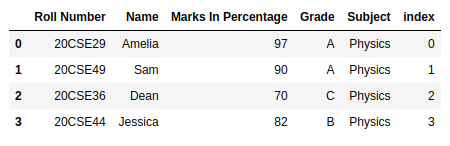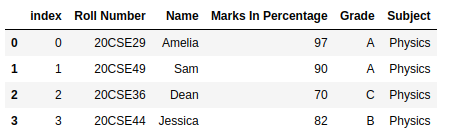# How to Convert Index to Column in Pandas Dataframe?

Pandas is a powerful tool which is used for data analysis and is built on top of the python library. The Pandas library enables users to create and manipulate dataframes (Tables of data) and time series effectively and efficiently. These dataframes can be used for training and testing machine learning models and Analyzing data.

## Converting Index to Columns

By default, each row of the dataframe has an index value. The rows in the dataframe are assigned index values from 0 to the (number of rows – 1) in a sequentially order with each row having one index value. There are many ways to convert an index to a column in a pandas dataframe. Let’s create a dataframe.

## Python3

 `# importing the pandas library as pd ` `import` `pandas as pd ` ` `  ` `  `# Creating the dataframe df ` `df ``=` `pd.DataFrame({``'Roll Number'``: [``'20CSE29'``, ``'20CSE49'``, ``'20CSE36'``, ``'20CSE44'``], ` `                   ``'Name'``: [``'Amelia'``, ``'Sam'``, ``'Dean'``, ``'Jessica'``], ` `                   ``'Marks In Percentage'``: [``97``, ``90``, ``70``, ``82``], ` `                   ``'Grade'``: [``'A'``, ``'A'``, ``'C'``, ``'B'``], ` `                   ``'Subject'``: [``'Physics'``, ``'Physics'``, ``'Physics'``, ``'Physics'``]}) ` ` `  `# Printing the dataframe ` `df`

Output:Method 1: The simplest method is to create a new column and pass the indexes of each row into that column by using the Dataframe.index function.

## Python3

 `import` `pandas as pd ` ` `  ` `  `df ``=` `pd.DataFrame({``'Roll Number'``: [``'20CSE29'``, ``'20CSE49'``, ``'20CSE36'``, ``'20CSE44'``], ` `                   ``'Name'``: [``'Amelia'``, ``'Sam'``, ``'Dean'``, ``'Jessica'``], ` `                   ``'Marks In Percentage'``: [``97``, ``90``, ``70``, ``82``], ` `                   ``'Grade'``: [``'A'``, ``'A'``, ``'C'``, ``'B'``], ` `                   ``'Subject'``: [``'Physics'``, ``'Physics'``, ``'Physics'``, ``'Physics'``]}) ` ` `  `# Printing the dataframe ` `df[``'index'``] ``=` `df.index ` `df`

Output:Method 2: We can also use the Dataframe.reset_index function to convert the index as a column. The inplace parameter reflects the change in the dataframe to stay permanent.

## Python3

 `import` `pandas as pd ` ` `  ` `  `df ``=` `pd.DataFrame({``'Roll Number'``: [``'20CSE29'``, ``'20CSE49'``, ``'20CSE36'``, ``'20CSE44'``], ` `                   ``'Name'``: [``'Amelia'``, ``'Sam'``, ``'Dean'``, ``'Jessica'``], ` `                   ``'Marks In Percentage'``: [``97``, ``90``, ``70``, ``82``], ` `                   ``'Grade'``: [``'A'``, ``'A'``, ``'C'``, ``'B'``], ` `                   ``'Subject'``: [``'Physics'``, ``'Physics'``, ``'Physics'``, ``'Physics'``]}) ` ` `  `# Printing the dataframe ` `df.reset_index(level``=``0``, inplace``=``True``) ` `df`

Output:My Personal Notes arrow_drop_upCheck out this Author's contributed articles.

If you like GeeksforGeeks and would like to contribute, you can also write an article using contribute.geeksforgeeks.org or mail your article to contribute@geeksforgeeks.org. See your article appearing on the GeeksforGeeks main page and help other Geeks.

Please Improve this article if you find anything incorrect by clicking on the "Improve Article" button below.

Article Tags :

Be the First to upvote.

Please write to us at contribute@geeksforgeeks.org to report any issue with the above content.